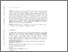# Kolmogorov’s Equations for Jump Markov Processes with Unbounded Jump Rates

Feinberg, E and Mandava, M and Shiryaev, A N (2017) Kolmogorov’s Equations for Jump Markov Processes with Unbounded Jump Rates. Annals of Operations Research.Preview
| Preview

## Abstract

As well-known, transition probabilities of jump Markov processes satisfy Kolmogorov’s backward and forward equations. In the seminal 1940 paper, William Feller investigated solutions of Kolmogorov’s equations for jump Markov processes. Recently the authors solved the problem studied by Feller and showed that the minimal solution of Kolmogorov’s backward and forward equations is the transition probability of the corresponding jump Markov process if the transition rate at each state is bounded. This paper presents more general results. For Kolmogorov’s backward equation, the sufficient condition for the described property of the minimal solution is that the transition rate at each state is locally integrable, and for Kolmogorov’s forward equation the corresponding sufficient condition is that the transition rate at each state is locally bounded.

ISB Creators:
ISB CreatorsORCiD
Mandava, Mhttps://orcid.org/0000-0002-3920-7805
Item Type: Article
Uncontrolled Keywords: Jump Markov process, Kolmogorov’s equation, minimal solution, boundedness condition, transition function, unbounded transition ratesView Item